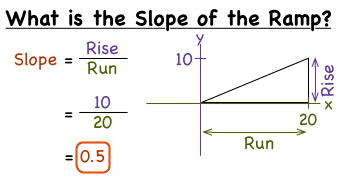Finding the slope of a ramp Math Lessons

# Finding the slope of a ramp Math LessonsFinding the slope of a ramp Math Lessons

Video covers how to find the slope of a ramp. You will often find the format for describing a ramp as a ratio. The video explains what a 1:12 ratio means and 1:16 ratio and how to apply this to finding the slpe of a ramp, and what the dimensions of the ramp should be.
Related Topics: